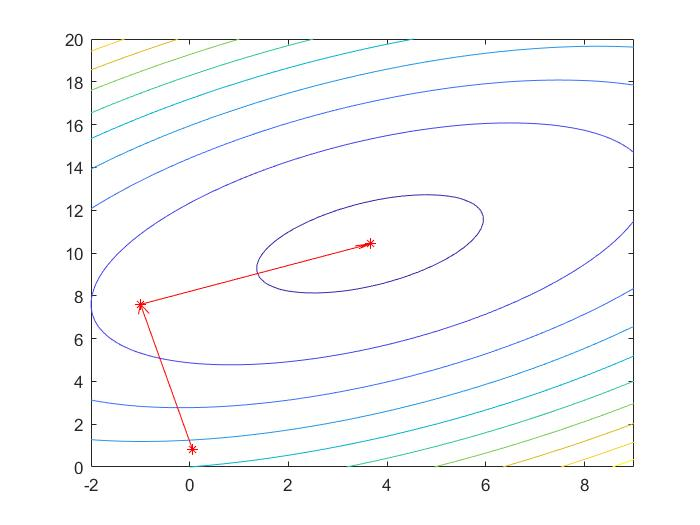• import numpy as np Q=np.matrix([[1,-0.5],[-0.5,1]]) b=np.matrix([[-1.56],[8.6]]) def F(x): global Q,b return 0.5*x.T*Q*x-x.T*b def g(x): return Q*x-b i=0 x0=np.random.rand(2,1) ...while i
import numpy as np

Q=np.matrix([[1,-0.5],[-0.5,1]])
b=np.matrix([[-1.56],[8.6]])

def F(x):
global Q,b
return 0.5*x.T*Q*x-x.T*b

def g(x):
return Q*x-b

i=0
x0=np.random.rand(2,1)
x=x0
d=g(x)
epsilon=1e-32
print("step 0 :",x.flatten())
while i<2:
alpha=-g(x).T*d/(d.T*Q*d+epsilon)
x=x+alpha[0,0]*d
beta=g(x).T*Q*d/(d.T*Q*d+epsilon)
d=-g(x)+beta[0,0]*d
i=i+1
print("step",i,":",x.flatten())展开全文算法
• 全波形反演是一种全新的地震...同时,应用共轭梯度法对正、逆断层模型和Marmousi模型进行了速度结构反演。反演结果表明:共轭梯度法计算效率较高,反演得到的速度模型精度更高,反演效果较好,是一种有效的波形反演方法。
• 主要介绍了python实现的共轭梯度法，文中通过示例代码介绍的非常详细，对大家的学习或者工作具有一定的参考学习价值，需要的朋友们下面随着小编来一起学习学习吧
• 为了寻找同时具有良好的收敛性和数值表现的共轭梯度法.将CD方法和LS方法结合,选用推广的Wolfe线搜索,构造出一类新的混合共轭梯度法.新的混合共轭梯度法不需要限制推广的Wolfe线搜索条件中的参数,但得到的下降性与CD...
• 为寻求收敛性质和数值表现具佳的无约束优化算法,利用共轭梯度法和含有两个方向调控参数的谱共轭梯度法,结合LS方法与CD方法给出混合的共轭参数和相应的谱参数,建立采用标准Wolfe线搜索的谱共轭梯度算法,证明了算法...
• matlab共轭梯度法求目标函数的最小极值-共轭梯度-王.rar 我是地球物理专业的一名学生，把自己实习的作业发上来大家分享下matlab
• 资源包括两个算法的python实现，分别是共轭梯度法和BFGS法，利用Numpy与Sympy，实现了只需输入函数与初始点等基本条件，即可求解并输出迭代过程种各参数变化。
• 针对无约束优化问题,提出一类谱共轭梯度法.谱共轭梯度法是对TS、GN及MPRP方法的修正,使得在任何线性搜索条件下都具有充分下降性.并且在Armijo型线性搜索条件下,证明了该类算法的全局收敛性.与GN、SFR及MPRP方法进行...
• 求解线性⽅方程组 Ax=b，其中 A 为 ...比较 Jacobi 迭代法、Gauss-Seidel 迭代法、逐次超松弛迭代法、 共轭梯度法与高斯消去法、列主元消去法的计算时间。改变逐次超松弛迭代法的松弛因⼦， 分析其对收敛速度的影响。
• 为了构造具有更好收敛性的谱共轭梯度法,根据已有的共轭系数β~*_k和β■,构造了一个新的共轭系数β■,从而给出了一个新的谱共轭梯度法。经过选取适当的谱系数,保证新方法在每次迭代时总能产生充分下降的搜索方向。该...
• FR共轭梯度法，输入目标函数、初始点、精度，能够得到整个求解过程，每一步迭代的结果都能打印出来，方便初学者学习，跟教材完全对应。
• 在本文中，我们基于Broyden-Fletcher-Goldfarb-Shanno（BFGS）方法的修正割线方程和新的改进的非单调线搜索技术，提供并分析了一种新的按比例缩放的共轭梯度法及其性能。 该方法合并了修改后的BFGS正割方程，以包括...
• 针对目前焦炭质量预测线性方法的现状及不足,建立了共轭梯度法优化的BP神经网络焦炭质量预测模型。该模型既能满足焦炭质量预测的非线性关系,又克服了神经网络收敛速度低、易陷入局部极小值的缺点。模型的实例验证分析...
• 提出了一种分析频谱的新方法，其主要思想是采用共轭梯度法训练傅里叶基神经网络权值，根据权值获得信号的幅度谱和相位谱，并给出了基于Matlab语言的频谱分析应用实例。仿真结果表明，与FFT相比，该方法具有计算精度...频谱分析
• 为寻求同时具有良好的收敛性和数值表现的共轭梯度法,在Wolfe线搜索下,构造一种修正的DY共轭梯度法.该算法产生的搜索方向为充分下降方向,这一性质与所采用的线搜索方法无关.在Wolfe线搜索的条件下证明该算法具全局...
• 基于DY和DL共轭梯度法,给出一个新的βk公式,在精确线搜索下该公式等价于βDkY.基于新参数公式建立了采用Wolfe线搜索的共轭梯度算法,证明了算法满足充分下降性和全局收敛性,初步的数值试验结果表明该方法是有效的,...
共轭梯度法

共轭方向法中，最大的问题在于寻找一组彼此独立的向量u1,...,un${u}_{1},...,{u}_{n}$$u_1,...,u_n$，如果选取不当，那么和高斯消元法就没有区别了。共轭梯度法 (Conjugate Gradient)实际上是一种特殊的共轭方向法，它取ui=r(i)${u}_{i}={r}_{\left(i\right)}$$u_i = r_{(i)}$。

首先为何残差是彼此独立的？首先，由于共轭方向法每次（A正交地）消除了一个维度上的误差，所以有
dTiAe(j)=0(i<j)${d}_{i}^{T}A{e}_{\left(j\right)}=0\left(i
d_i^T A e_{(j)} = 0 (i<j)

由于r(j)=−Ae(j)${r}_{\left(j\right)}=-A{e}_{\left(j\right)}$$r_{(j)} = - A e_{(j)}$，于是有dTir(j)=0${d}_{i}^{T}{r}_{\left(j\right)}=0$$d_i^T r_{(j)} = 0$。另外，由于现在的ui=r(i)${u}_{i}={r}_{\left(i\right)}$$u_i = r_{(i)}$，所以
span{d1,...,dn}=span{ui,...,un}=span{r(1),...,r(n)}$span\left\{{d}_{1},...,{d}_{n}\right\}=span\left\{{u}_{i},...,{u}_{n}\right\}=span\left\{{r}_{\left(1\right)},...,{r}_{\left(n\right)}\right\}$
span\{d_1,...,d_n\} = span\{u_i,...,u_n\} = span\{r_{(1)},...,r_{(n)}\}

r(j)${r}_{\left(j\right)}$$r_{(j)}$和di(i<j)${d}_{i}\left(i$d_i(i张成的子空间正交，因此也和所有的r(i)(i<j)${r}_{\left(i\right)}\left(i$r_{(i)}(i正交。

共轭梯度法和最速下降法的区别

这里我产生的一个疑问是，残差其实就是梯度的（反）方向，既然当次迭代的梯度方向总是和之前的梯度方向垂直，那么它和最速下降法有什么区别？为何最速下降法会出现“之”字形的迭代路线？我认为是这样的。首先，在最速下降法中，当次迭代的梯度方向也是和上次迭代梯度方向垂直，但和再之前的梯度方向就不垂直了，所以会有“之”形路线。然后，共轭梯度法要求的是关于矩阵正交，并非直接正交。在上一节的图中，我们可以看到关于矩阵正交的形状，通过拉伸，可以看到，它才能真正的分离维度，普通的正交反而是有偏差的。共轭方向法将本来普通正交的梯度向量，调整为新的关于矩阵正交，虽然张成的空间是一样的，但方向更加合理，所以能保证在n$n$$n$步内迭代收敛。

共轭梯度法的优势

于是我们可以看到，起码共轭梯度法作为一种特殊的共轭方向法，是没有错误的。那共轭梯度法有什么优势呢？

先来看一个有趣的性质。在上一节的末尾，我们提到了迭代更新残差的方法：
r(i+1)=r(i)−α(i)Adi${r}_{\left(i+1\right)}={r}_{\left(i\right)}-{\alpha }_{\left(i\right)}A{d}_{i}$
r_{(i+1)} = r_{(i)} - \alpha_{(i)} A d_i

注意到新的残差（或梯度）引入了一个新的维度（因为新的搜索方向和之前所有梯度张成的空间关于矩阵正交）。即假设span{r(0),...,r(i+1)}=D(i+1)$span\left\{{r}_{\left(0\right)},...,{r}_{\left(i+1\right)}\right\}={D}_{\left(i+1\right)}$$span\{r_{(0)},...,r_{(i+1)}\}=D_{(i+1)}$，那么有rank(D(i+1))=rank(D(i))+1$rank\left({D}_{\left(i+1\right)}\right)=rank\left({D}_{\left(i\right)}\right)+1$$rank(D_{(i+1)}) = rank(D_{(i)}) + 1$。显然r(i)∈D(i)${r}_{\left(i\right)}\in {D}_{\left(i\right)}$$r_{(i)} \in D_{(i)}$，新维度只能来源于Adi$A{d}_{i}$$Ad_i$，而di∈D(i)${d}_{i}\in {D}_{\left(i\right)}$$d_i \in D_{(i)}$，所以A$A$$A$带来的变换引入了新维度。引申开来，不难得到
D(i)=span{d1,...,dn}=span{d1,Ad1,A2d1,...,Ai−1d1}=span{r(1),Ar(1),A2r(1),...,Ai−1r(1)}${D}_{\left(i\right)}=span\left\{{d}_{1},...,{d}_{n}\right\}=span\left\{{d}_{1},A{d}_{1},{A}^{2}{d}_{1},...,{A}^{i-1}{d}_{1}\right\}=span\left\{{r}_{\left(1\right)},A{r}_{\left(1\right)},{A}^{2}{r}_{\left(1\right)},...,{A}^{i-1}{r}_{\left(1\right)}\right\}$
D_{(i)} = span\{d_1,...,d_n\} = span\{d_1, Ad_1, A^2d_1, ..., A^{i-1}d_1\} = span\{r_{(1)}, Ar_{(1)}, A^2r_{(1)}, ..., A^{i-1}r_{(1)}\}

诸如此类的，由一个向量反复乘以同样的矩阵变换张成的子空间被成为克雷洛夫子空间 (Krylov Subspace)。这样的子空间有一个很好的性质，那就是施密特正交化法会变得非常容易。

在上一节中，我们给出了施密特正交化法的参数表达式，
βij=r(i)AdjdTjAdj${\beta }_{ij}=\frac{{r}_{\left(i\right)}A{d}_{j}}{{d}_{j}^{T}A{d}_{j}}$

由于
rT(i)r(j+1)=rT(i)(r(j)−α(j)Adj)=rT(i)r(j)−α(j)rT(i)AdjrT(i)Adj=1α(j)[rT(i)r(j)−rT(i)r(j+1)]${r}_{\left(i\right)}^{T}{r}_{\left(j+1\right)}={r}_{\left(i\right)}^{T}\left({r}_{\left(j\right)}-{\alpha }_{\left(j\right)}A{d}_{j}\right)={r}_{\left(i\right)}^{T}{r}_{\left(j\right)}-{\alpha }_{\left(j\right)}{r}_{\left(i\right)}^{T}A{d}_{j}\phantom{\rule{0ex}{0ex}}{r}_{\left(i\right)}^{T}A{d}_{j}=\frac{1}{{\alpha }_{\left(j\right)}}\left[{r}_{\left(i\right)}^{T}{r}_{\left(j\right)}-{r}_{\left(i\right)}^{T}{r}_{\left(j+1\right)}\right]$
r_{(i)}^T r_{(j+1)} =	r_{(i)}^T (r_{(j)} - \alpha_{(j)} A d_j) = r_{(i)}^T r_{(j)} - \alpha_{(j)} r_{(i)}^T A d_j
\\ r_{(i)}^T A d_j = \frac{1}{\alpha_{(j)}} [ r_{(i)}^T r_{(j)} - r_{(i)}^T r_{(j+1)} ]

由于i>j$i>j$$i>j$，所以
(i=j+1):βij=−1α(j)rT(i)r(i)dTjAdj(i>j+1):βij=0$\left(i=j+1\right):{\beta }_{ij}=-\frac{1}{{\alpha }_{\left(j\right)}}\frac{{r}_{\left(i\right)}^{T}{r}_{\left(i\right)}}{{d}_{j}^{T}A{d}_{j}}\phantom{\rule{0ex}{0ex}}\left(i>j+1\right):{\beta }_{ij}=0$
(i=j+1): \beta_{ij} = - \frac{1}{\alpha_{(j)}} \frac{r_{(i)}^T r_{(i)}}{d_j^TAd_j}
\\ (i>j+1): \beta_{ij} = 0

这样，共轭梯度法作为一种特殊的共轭方向法，解决了施密特正交化法速度慢的问题。当然α(j)${\alpha }_{\left(j\right)}$$\alpha_{(j)}$的表达式和之前是一致的，即
α(j)=dTjr(j)dTjAdj${\alpha }_{\left(j\right)}=\frac{{d}_{j}^{T}{r}_{\left(j\right)}}{{d}_{j}^{T}A{d}_{j}}$
\alpha_{(j)} = \frac{d_j^T r_{(j)}}{d_j^T A d_j}

代入α(j)${\alpha }_{\left(j\right)}$$\alpha_{(j)}$，得到
βi=βi,i−1=−rT(i)r(i)dTi−1r(i−1)=−rT(i)r(i)rT(i−1)r(i−1)${\beta }_{i}={\beta }_{i,i-1}=-\frac{{r}_{\left(i\right)}^{T}{r}_{\left(i\right)}}{{d}_{i-1}^{T}{r}_{\left(i-1\right)}}=-\frac{{r}_{\left(i\right)}^{T}{r}_{\left(i\right)}}{{r}_{\left(i-1\right)}^{T}{r}_{\left(i-1\right)}}$
\beta_i = \beta_{i,i-1} = - \frac{r_{(i)}^T r_{(i)}}{d_{i-1}^T r_{(i-1)}} = - \frac{r_{(i)}^T r_{(i)}}{r_{(i-1)}^T r_{(i-1)}}

展开全文优化方法
• 当复线性方程组的规模较大或系数矩阵的条件数很大时，系数矩阵易呈现病态特性，双共轭梯度法存在不收敛和收敛速度慢的潜在问题，采用适当的预处理技术，可以改善矩阵病态特性，加快收敛速度。从实型不完全Cholesky...
• 在这里，共轭梯度法用于解决基于模型的改进的最优控制问题，其中反复使用所用模型的最优解，然后在每个迭代步骤中更新调整后的参数。 当达到收敛时，尽管模型与现实之间存在差异，但迭代解决方案仍将逼近原始最优...
• 本文对迭代盲目去卷积复原方法进行了研究，提出了基于频域共轭梯度法的交替迭代优化复原算法，在频域上构造了关于目标图像和点扩展函数频谱的误差代价函数，将共轭梯度法引入到频谱误差代价函数的极小化过程中，并将...
• main为主函数 fun gfun ggfun分别为输入的...分别为最速下降法 牛顿法（阻尼）共轭梯度法 以及 拟牛顿法 F1-4为下降的图示 可以看到牛顿法和拟牛顿法收敛速度最快 但是牛顿法需要求矩阵的逆 在实际中 运算量可能较大...